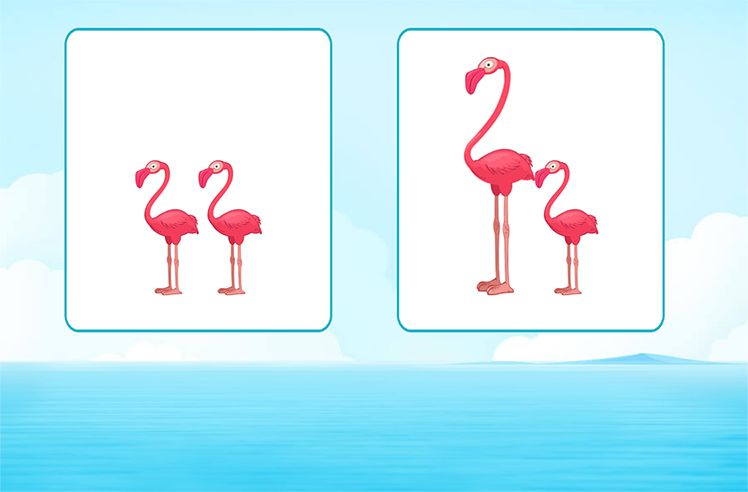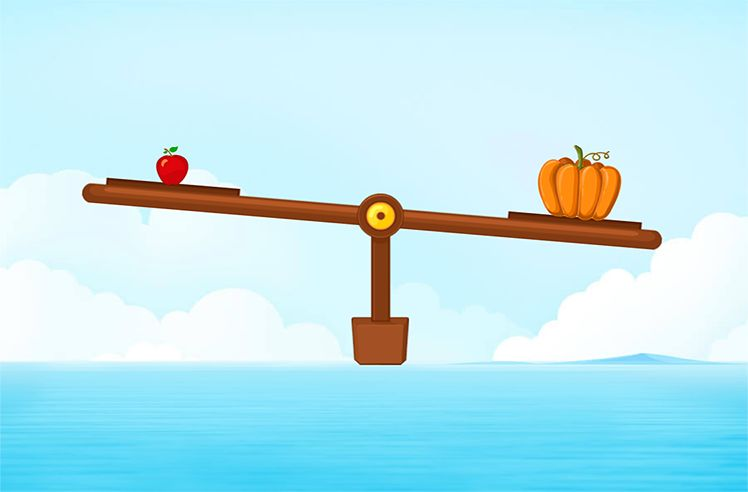# Scale (On A Graph) – Definition with Examples

» Scale (On A Graph) – Definition with Examples

## Scale on a Graph

Data

Data is the collected facts. We often need to find things such as:

• The population of a town
• Number of students in a school
• Sales of a particular product during a week
• Likings of people, etc

In such cases, we collect data. We read and interpret the collected data to make sense of it. One of the ways of showing the processed data is through graphs.

A graph is a visual representation of data.

Some common graphs are:

We use a scale to measure or quantify objects. Let us understand what a scale is with the help of a pictograph.

The above pictograph shows the information for the favorite musical instrument of children. Can you tell how many children like Guitar or Drum or Keyboard?

What does 1represent?

Well, it can be of any value. But unless and until we don’t show it on the graph, we won’t be able to tell the exact number of people. In such cases, we use a scale.

Now, we know, 1represents 5 children. So, now we can say, 15 children like guitar, 10 like the drum and 20 like the keyboard.

Scale

In simple words, a scale is a set of numbers that help to measure or quantify objects. A scale on the graph shows the way the numbers or pictures are used in data.

Let us now move on to a bar graph. We use the same data of “Favorite Musical Instrument” to plot a Bar graph.

• Here, the vertical axis (y-axis), shows the number of children and the horizontal axis (x-axis) shows the musical instruments.
• On the y-axis, the numbers are marked at intervals of 5. This indicates that the scale used for the graph is 1 unit is 5 children.

The distance between two numbers indicates a unit and this unit remains uniform throughout a scale. Thus, a scale plays a crucial part in plotting graphs. Without scales, we won’t be able to infer anything relevant from the graph.Comparing Lengths

Play NowComparing Heights

Play NowComparing Weights

Play Now